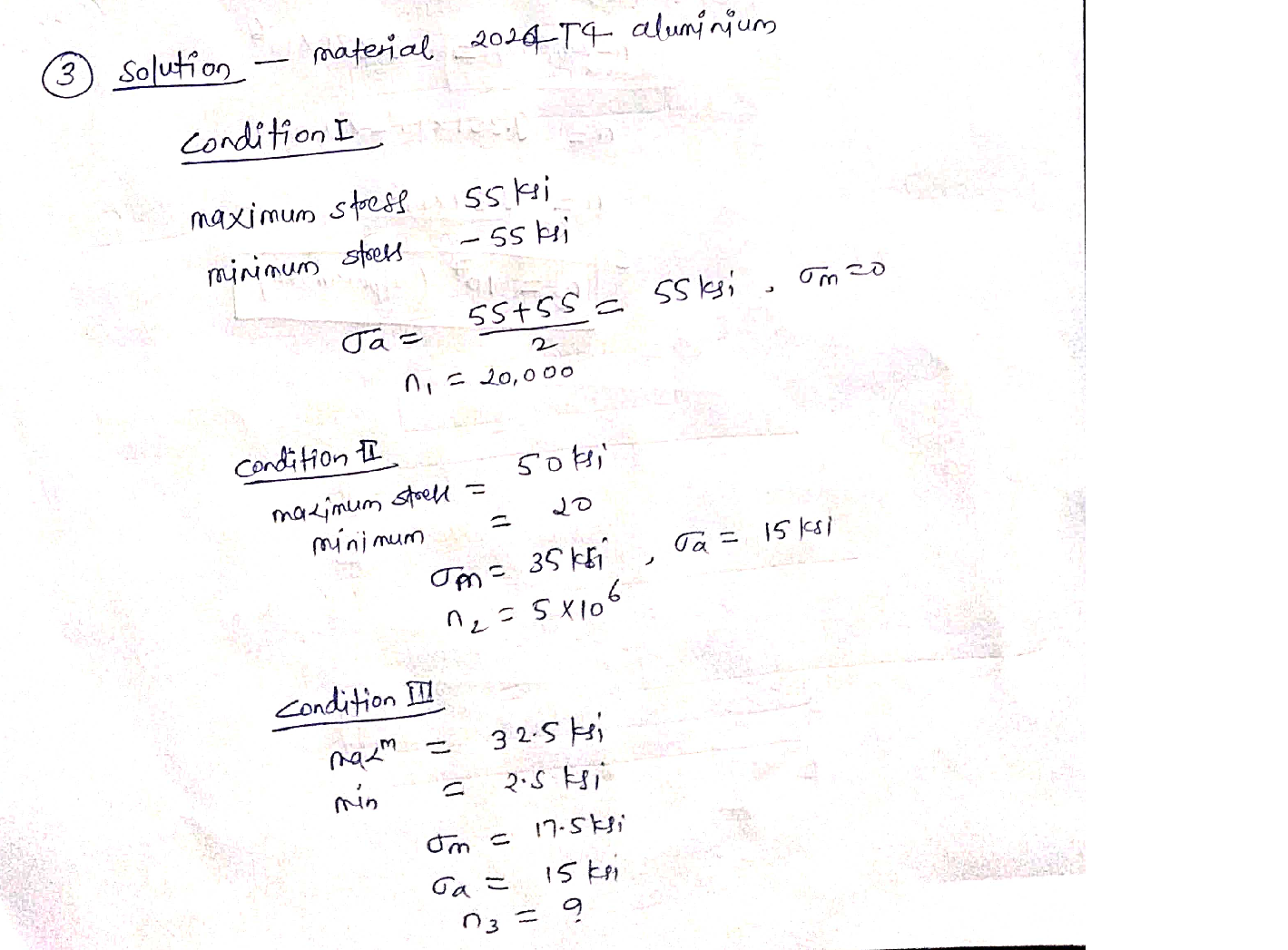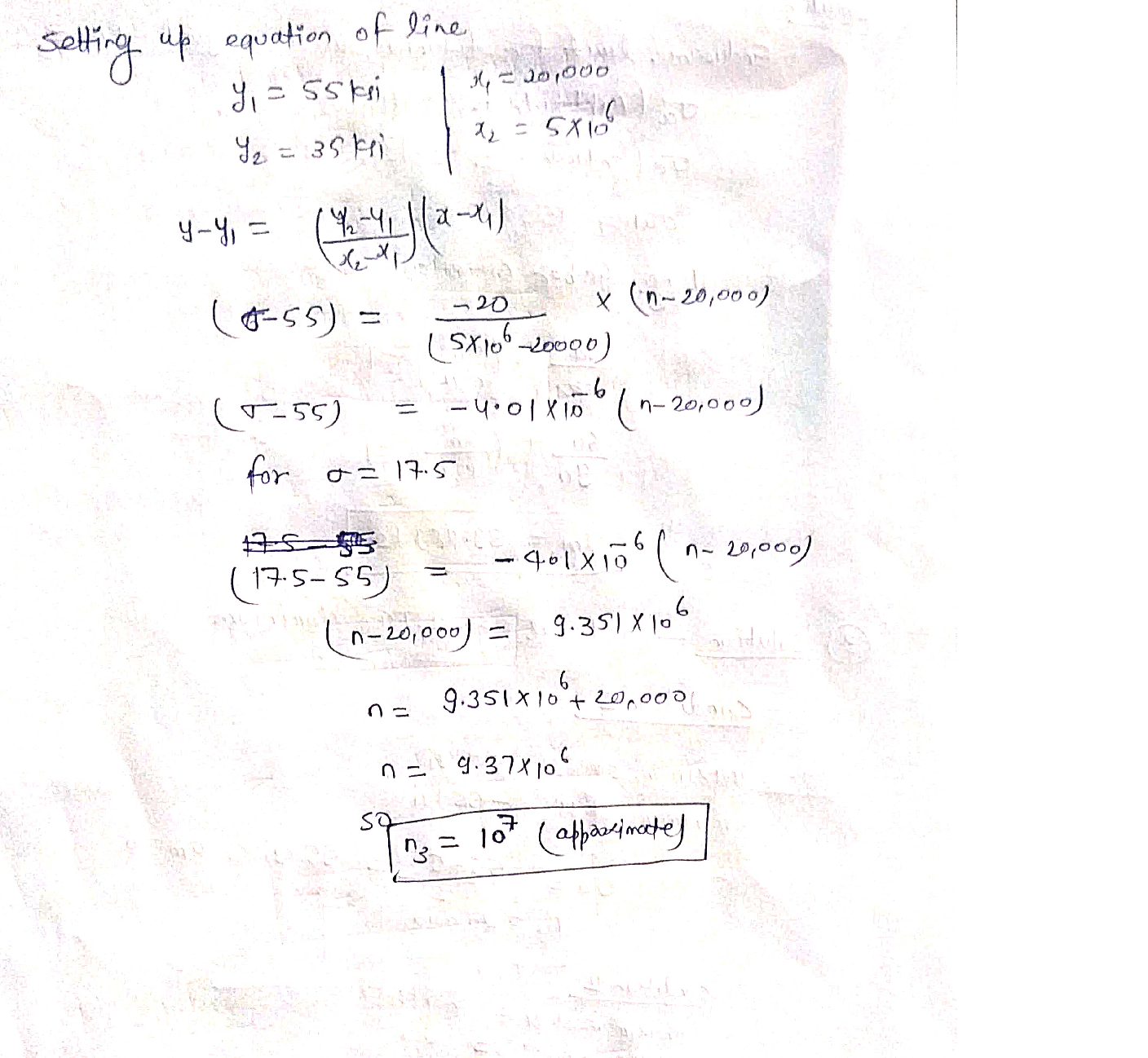Question

Material Science

S. A chassis support member made from 2024-T4 aluminum accumulates the following cyclic stress histories:

Find the number of cycles n; for the stress condition 3 that the member can accumulate after experiencing the cyclic stress history of stress conditions 1 and 2. Base all calculations on 50 percent survival rates. Note: os=70 ksiVerified### Question 45724Material Science

Q#8. The plot in Figure 5 shows the creep data for tungsten at 2400 °C for various stress (o) levels. If the dependence of creep rate on stress is given by A", calculate n. (5 points)

### Question 45723Material Science

. Consider the Cu-Zn phase diagram in Figure 4 (10 points)
(a) What is the maximum solid solubility in weight percent of Zn in Cu in the terminal solid solution a?
(b) Identify the intermediate phases in the Cu-Zn phase diagram.
(c) Identify the eutectoid reactions in the Cu-Zn diagram.
(1) Determine the composition and temperature coordinates of the reactions.
(ii) Write the equations for the reactions.

### Question 45722Material Science

Q#6. Consider the binary eutectic copper-silver phase diagram in Figure 3. Make phase analyses of an 88 wt %Ag-12 wt % Cu alloy at the temperatures. (10 points)
(а) 1000°C
(b) 800°C
(c) 780°C + AT , and
(d) 780°C - AT.
In the phase analyses, include:
(ii) The chemical compositions of the phases
(iii) The amounts of each phase
(iv) Sketch the microstructure by using 2 cm diameter circular fields.

### Question 45721Material Science

Q#5. Write equations for the following invariant reactions: eutectic, eutectoid, peritectic, and peritectoid. How many degrees of freedom exist at invariant reaction points in binary phase diagrams? (5 points)

### Question 45720Material Science

Q#4. Consider the single crystal iron rod given in the Figure 2. The diameter of the rod is 12 mm. The rod is Tobe uniaxially loaded as shown. If the critical resolved shear stress for the slip system given is 45 MPa, what will be the uniaxial force, F, to initiate yielding? (5 points)

### Question 45719Material Science

Q#3. The diffusivity of iron atoms in the BCC iron lattice is 4.5 x 1023 m²/s at 400°C and 5.9 × 10-16 m²/s at800°C. Calculate the activation energy in kJ/mol for this case in this temperature range. R = 8.314 J/(mol · K).(5 noints)

### Question 45718Material Science

Q#2. A metal wire of 50 cm length and 1 mm radius is exposed to a sequence of loading and unloading steps as described below. The stress-strain data for the metal is given in Figure 1. (5 points)
a) What is the total length of the wire in Step 1.
c) What is the total length in Step 3?
b) What is the total length in Step 2?
d) What is the final yield strength?

### Question 45717Material Science

Q#1. A fully stabilized, cubic polycrystalline ZrO2 sample has a fracture toughness of Kic=3.8 MPa – /m when tested on a four-point bend test. (5 points)
(a) If the sample fails at a stress of 450 MPa, what isthe size of the largest surface flaw? Assume Y = Vn.
(b) The same test is performed with a partially stabilized ZrO2 specimen. This material is transformation-toughened and has a Kic = 12.5MPa-Vm. If this material has the same flaw distribution as the fully stabilized sample, what stress must be applied to cause failure?

### Question 44863Material Science

The company you work for produces a Titanium alloy with two different grain sizes to get two different strength values. The yield strength is 100 MPa for an annealed alloy with a grain diameter, d = 400 um. Ifyou cold work and recrystallize the alloy to achieve grain diameter, d = 4 um, the yield strength is increased to 280 MPa.
After reading the "Over Simplified and Sensationalized Mechanics for People without Scientific Backgrounds" magazine your boss is very excited about A.I., nanotechnology, robots, and drones. Now he is interested in producing nano-structured Titanium exoskeletons for some flying sentient weaponized cockroach-like "toys" he wants to develop for a mysterious and secretive client who lives in a volcano on a private island. He asks you to determine the strength of this Titanium alloy if you can reduce the grain diameter to 100 nanometers.
Determine the Hall-Petch constants:
\sigma_{0}=
k=\quad M P a \cdot \sqrt{m}
Calculate the possible yield strength of this alloy with a grain diameter of 100 nanometers:

### Question 44862Material Science

Using the phase diagram and the aging curves on the equation sheet for aluminum alloyed with copperplease answer the following.
a) Over what composition range (in wt% Cu), can you produce Al alloys precipitation hardened with Cu? Beas specific as possible. (2points)
c) Explain why the material's strength starts to decrease if you age it for too long. (2 points)
b) Supposing you have an Al alloy with 4 wt% Cu, describe in as much detail as possible EACH STEP(assuming previous processing unknown) of the procedure you need to use in order to achieve a combination aof tensile strength greater than 400 MPa and ductility of less than 10% elongation. Give detailed temperature and time where necessary. There could be multiple possible answers, give only one answer. (8 points)

### Submit query